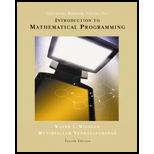## Solutions for Introduction to mathematical programming

### Book Details

1. INTRODUCTION TO MODEL BUILDING.

An Introduction to Modeling.
The Seven-Step Model-Building Process.
Examples.

2. BASIC LINEAR ALGEBRA.

Matrices and Vectors.
Matrices and Systems of Linear Equations.
The Gauss-Jordan Method for Solving Systems of Linear Equations.
Linear Independence and Linear Dependence.
The Inverse of a Matrix.
Determinants.

3. INTRODUCTION TO LINEAR PROGRAMING.

What is a Linear Programming Problem? The Graphical Solution of Two-Variable Linear Programming Problems.
Special Cases.
A Diet Problem.
A Work-Scheduling Problem.
A Capital Budgeting Problem. Short-term Financial Planning.
Blending Problems.
Production Process Models.
Using Linear Programming to Solve Multiperiod Decision Problems: An Inventory Model.
Multiperiod Financial Models.
Multiperiod Work Scheduling.

4. THE SIMPLEX ALGORITM AND GOAL PROGRAMING.

How to Convert an LP to Standard Form.
Preview of the Simplex Algorithm. The Simplex Algorithm.
Using the Simplex Algorithm to Solve Minimization Problems.
Alternative Optimal Solutions. Unbounded LPs.
The LINDO Computer Package.
Matrix Generators, LINGO, and Scaling of LPs.
Degeneracy and the Convergence of the Simplex Algorithm.
The Big M Method.
The Two-Phase Simplex Method.
Unrestricted-in-Sign Variables.
Karmarkar?s Method for Solving LPs.
Multiattribute Decision-Making in the Absence of Uncertainty: Goal Programming.
Solving LPs with Spreadsheets.

5. SENSITIVITY ANALYSIS: AN APPLIED APPROACH.

A Graphical Introduction to Sensitivity Analysis.
The Computer and Sensitivity Analysis.
Managerial Use of Shadow Prices.
What Happens to the Optimal z-value if the Current Basis is no Longer Optimal?

6. SENSITIVITY ANALYSIS AND DUALITY.

A Graphical Introduction to Sensitivity Analysis.
Some Important Formulas.
Sensitivity Analysis.
Sensitivity Analysis When More Than One Parameter is Changed: The 100% Rule.
Finding the Dual of an LP.
Economic Interpretation of the Dual Problem.
The Dual Theorem and Its Consequences.
Duality and Sensitivity Analysis.

7. TRANSPORTATION, ASSIGNMENT, AND TRANSSHIPMENT PROBLEMS.

Formulating Transportation Problems.
Finding Basic Feasible Solutions for Transportation Problems.
The Transportation Simplex Method.
Sensitivity Analysis for Transportation Problems.
Assignment Problems.
Transshipment Problems.

8.NETWORK MODELS.

Basic Definitions.
Shortest Path Problems.
Maximum Flow Problems.
CPM and PERT.
Minimum Cost Network Flow Problems.
Minimum Spanning Tree Problems.
The Network Simplex Method.

9. INTEGER PROGRAMMING.

Introduction to Integer Programming.
Formulation Integer Programming Problems.
The Branch-and-Bound Method for Solving Pure Integer Programming Problems.
The Branch-and-Bound Method for Solving Mixed Integer Programming Problems. Solving Knapsack Problems by the Branch-and-Bound Method.
Solving Combinatorial Optimization Problems by the Branch-and-Bound Method.
Implicit Enumeration.
The Cutting Plane Algorithm.

10. ADVANCED TOPICS IN LINEAR PROGRAMMING.

The Revised Simplex Algorithm.
The Product Form of the Inverse.
Using Column Generation to Solve Large-Scale LPs.
The Dantzig-Wolfe Decomposition Algorithm.
The Simplex Method for Upper-Bounded Variables.
Karmarkar?s Method for Solving LPs.

11. GAME THEORY.

Two-Person Zero-Sum and Constant-Sum Games: Saddle Points.
Two-Person Zero-Sum Games: Randomized Str4ategies, Domination, and Graphical Solution.
Linear Programming and Zero-Sum Games.
Two-Person Nonconstant-Sum Games.
Introduction to n-Person Game Theory.
The Core o f an n-Person Game.
The Shapely Value.

12. NONLINEAR PROGRAMMING

Review of Differential Calculus.
Introductory Concepts.
Convex and Concave Functions.
Solving NLPs with One Variable.
Golden Section Search.
Unconstrained Maximization and Minimization with Several Variables.
The Method of Steepest Ascent. Lagrange Multiples.
The Kuhn-Tucker Conditions.
Separable Programming.
The Method of Feasible Directions.
Pareto Optimality and Tradeoff Curves.

13. DETERMINISTIC DYNAMIC PROGRAMMING.

Two Puzzles.
A Network Problem.
An Inventory Problem.
ResourceAllocation Problems.
Equipment Replacement Problems.
Formulation Dynamic Programming Recursions.
Using Spreadsheets to Solve Dynamic Programming Problems.

14. HEURISTIC METHODS.

15. NEURAL NETWORKS.

Answers To Selected Problems.
Index.

## Sample Solutions for this Textbook

We offer sample solutions for Introduction to mathematical programming homework problems. See examples below:

## More Editions of This Book

Corresponding editions of this textbook are also available below: